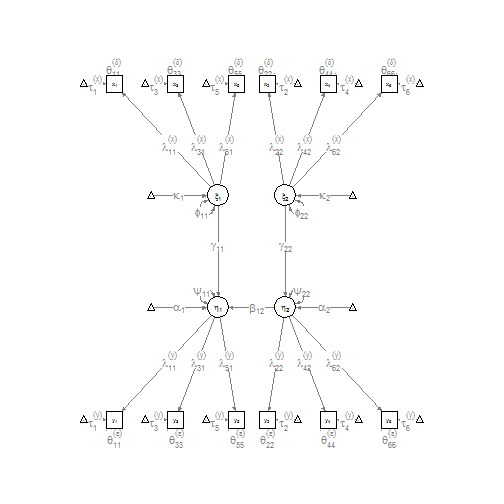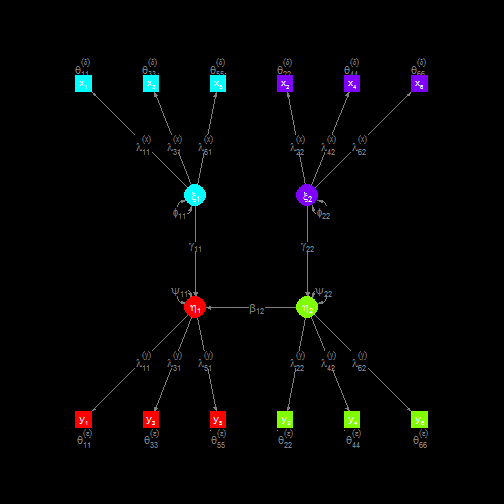lisrelModel {semPlot} R Documentation

Construct SEM model using LISREL matrix specification.

Description

This function creates a 'semPlotModel' object using matrices of the extended LISREL model (Joreskog & Sorbom, 1996). This function has two main purposes. First, it can be used to easilly create path diagrams of arbitrary SEM models without having to run an actual analysis. And second, it is specifically designed to work with the output of the 'lisrelToR' package (using `do.call(lisrelModel,output\$matrices)`). Using `semPaths` or `semPlotModel` on the file path of a LISREL output file will automatically first run `readLisrel` and then this function.

Usage

```lisrelModel(LY, PS, BE, TE, TY, AL, manNamesEndo, latNamesEndo, LX, PH, GA, TD, TX, KA, manNamesExo, latNamesExo, ObsCovs, ImpCovs, setExo, modelLabels = FALSE)
```

Arguments

 `LY` Specification of the Lambda-Y matrix. See details. `PS` Specification of the Psi matrix. See details. `BE` Specification of the Beta matrix. See details. `TE` Specification of the Theta-Epsilon matrix. See details. `TY` Specification of the Tau-Y matrix. See details. `AL` Specification of the Alpha matrix. See details. `manNamesEndo` Character vector of names for the endogenous manifests. `latNamesEndo` Character vector of names for the endogenous latents. `LX` Specification of the Lambda-X matrix. See details. `PH` Specification of the Phi matrix. See details. `GA` Specification of the Gamma matrix. See details. `TD` Specification of the Theta-Delta matrix. See details. `TX` Specification of the Tau-X matrix. See details. `KA` Kappa `manNamesExo` Character vector of names for the exogenous manifests. `latNamesExo` Character vector of names for the exogenous latents. `ObsCovs` The observed covariance matrix, or a list of such matrices for each group. `ImpCovs` The implied covariance matrix, or a list of such matrices for each group. `setExo` Logical. If TRUE the 'exogenous' variable in the Variables data frame is specified. This forces `semPaths` to not attempt to identify which variables are endogenous and exogenous. `modelLabels` Logical. If TRUE all labels are set to the LISREL model matrix terms, as expressions. When plotted with `semPaths` this requires the argument `as.expression=c("nodes","edges")`.

Details

The LISREL matrices can be assigned in various ways, depending on the amount of information that should be stored in the resulting model.

First, the a single matrix can be used. The values of this matrix correspond to the parameter estimates in the 'semPlotModel'. For multiple groups, a list of such matrices can be used.

to store more information, a named list of multiple matrices of the same dimensions can be used. Included in this list can be the following (but only estimates is nessesary):

`est`

Parameter estimates

`std`

standardized parameter estimates

`par`

Parameter numbers. 0 indicating fixed variables and parameters with the same parameter number are constrained to be equal.

`fixed`

Logical matrix indicating if the parameter is fixed.

If `std` is missing the function tries to compute standardized solutions (not yet working for intercepts). If `fixed` is missing it is computed from the `par` matrix. For multiple groups, a list containing such lists can be used.

The number of variables is extracted from the assigned matrices. Matrices that are not assigned are assumed to be empty matrices of the appropriate dimensions. e.g., Lambda-Y is assumed to be a 0 by 0 matrix if there are no endogenous variables.

Value

A 'semPlotModel' object.

Author(s)

Sacha Epskamp <mail@sachaepskamp.com>

References

Joreskog, K. G., & Sorbom, D. (1996). LISREL 8 user's reference guide. Scientific Software.

https://github.com/SachaEpskamp/lisrelToR

`semPlotModel` `semCors` `semPaths`

Examples

``````
## Example of a Full LISREL model path diagram with the same number of exgenous and endogenous variables:

# Lambda matrices:

# Phi and Psi matrices:
LatVar <- diag(1,2,2)

# Beta matrix:
Beta <- matrix(0,2,2)
Beta[1,2] <- 1

# Theta matrices:

# Gamma matrix:
Gamma <- diag(1,2,2)

# Tau matrices:
ManInts <- rep(1,6)

# Alpha and Kappa matrices:
LatInts <- rep(1,2)

# Combine model:
TY=ManInts,TX=ManInts,AL=LatInts,KA=LatInts)

# Plot path diagram:
semPaths(mod, as.expression=c("nodes","edges"), sizeMan = 3, sizeInt = 1, sizeLat = 4)
````````````
# Plot path diagram with more graphical options:
semPaths(mod, as.expression=c("nodes","edges"), sizeMan = 3, sizeInt = 1, sizeLat = 4, label.prop=0.5, curve=0.5, bg="black", groups="latents", intercepts=FALSE, borders=FALSE, label.norm="O")
````````````
``````

[Package semPlot version 0.3 Index]